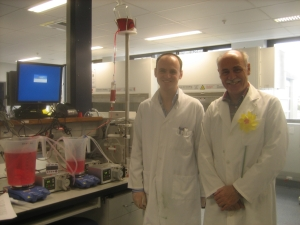Faculty of Medical and Health Sciences
Department of Pharmacology & Clinical Pharmacology, University of Auckland
Faculty of Medical and Health Sciences
Department of Pharmacology & Clinical Pharmacology, University of Auckland

# Two compartment beaker model

### Objective and introduction# Objectives

1. To observe the time course of drug concentration in the centrl and peripheral compartments of a two-compartment system.
2. To compare the time course after 3 types of input.
1. Single Bolus Dose
2. Single Bolus Dose and Constant Rate Infusion
3. Single Bolus Dose plus First-Order and Constant Rate Infusion

# Introduction

This is a practical session held in a laboratory. For health and safety reasons it is important to wear a lab coat and closed shoes.Bring a printed copy of the lab instructions and read through them before you arrive at the lab.

# Overview

1. Start the experiment by adding doses of amaranth dye to the beaker system.
2. Take samples from the central and tissue compartment beakers.
3. Measure the absorbance of the samples using a spectrophotometer.
4. Plot the time course of absorbance while the experiment is being performed,
5. Construct a standard curve relating absorbance to amaranth dye concentrations on two occasions.
6. Create a data set following the directions in the lab instructions.
7. Use either MONOLIX and the beka_mlxt model (Figure 1) or NONMEM and the beka.ctl model (Figure 2) to estimate the parameters of the two compartment system.
8. You will need to change the constants defining the 3 kinds of input in order to match the kinds of input you used for your experiment.

\$PROBLEM BEKA two compartment model with bolus, zero-order and first-order input

\$MODEL
COMP=(AMTC) ; central
COMP=(AMTT) ; tissue
COMP=(AMTB) ; burette

\$PSI
cl vc clic vt ; PK parameters

\$PK

; Check these values match the experimental design
bolus=10 ; 10 mg bolus dose into central compartment
infrate=1 ; 1 mg/min infusion into burette
burdose=40 ; 40 mg bolus dose into burette
burvol=0.1 ; 0.1 L burette volume
burflow=0.01 ; 0.01 L/min burette flow
tk0=120 ; 120 min infusion from burette

\$ODE
AMTC_0 = bolus
AMTT_0 = 0
AMTB_0 = infrate/burflow*burvol+burdose

CC=AMTC/vc
CT=AMTT/vt
CB=AMTB/burvol

;'t' is the name used by Monolix to

; refer to the data item defined as TIME.

if (t<tk0) then
ratein=burflow*CB
else
ratein=0
endif

DDT_AMTC = ratein + clic*CT - (cl+clic)*CC
DDT_AMTT = clic*(CC - CT)
DDT_AMTB = infrate - ratein

\$OUTPUT
OUTPUT1 = AMTC/vc
OUTPUT2 = AMTT/vt

Figure 1. Code for beka_mlxt.txt

\$PROBLEM BEKA two compartment model with bolus, zero-order and first-order input

\$INPUT ID TIME DVID ABS DV
\$DATA beka.csv
\$EST METHOD=COND INTER
MAX=9990 NSIG=3 SIGL=9 NOABORT PRINT=1

\$THETA
(0,0.1,) ; CL
(0,1,) ; VC
(0,0.4,) ; CLIC
(0,4,) ; VT

\$OMEGA 0 FIX ; PPV_CL
\$SIGMA
1 ; RUVC_SD
1 ; RUVT_SD

\$MODEL
COMP=(AMTC) ; central
COMP=(AMTT) ; tissue
COMP=(AMTB) ; burette

\$PK
bolus=10 ; 10 mg bolus dose into central compartment
infrate=1 ; 1 mg/min infusion into burette
burdose=40 ; 40 mg bolus dose into burette
burvol=0.1 ; 0.1 L burette volume
burflow=0.01 ; 0.01 L/min burette flow
tk0=120 ; minutes of infusion from burette

cl=CL*EXP(PPV_CL)

A_0(1) = bolus
A_0(2) = 0
A_0(3) = infrate/burflow*burvol+burdose

\$DES

CC=A(1)/vc
CT=A(2)/vt
CB=A(3)/burvol

; T is time used by differential equation solver
if (T<tk0) then
ratein=burflow*CB
else
ratein=0
endif

DADT(1) = ratein + clic*CT - (cl+clic)*CC

\$ERROR

CCONC = A(1)/vc
TCONC = A(2)/vt

if (dvid.eq.1) then
y=CCONC + RUVC_SD
else
y=TCONC + RUVT_SD
endif

\$TABLE ID TIME Y dvid